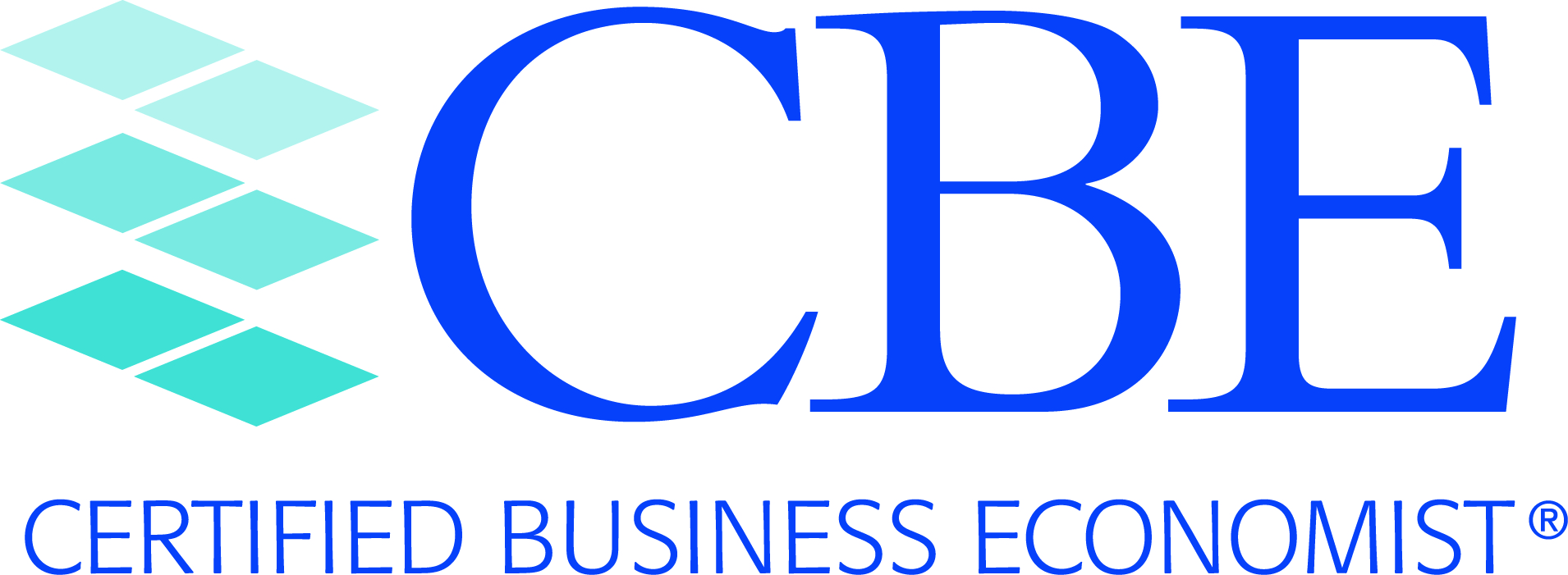Applied Econometrics - Content Outline

1. Getting Started
• The Model Specification Phase
• The Principal of Parsimony
• The Shrinkage Principle
• The Generic Single-Equation Linear Regression Model
• Components of the Model
• Endogenous Variables, Exogenous Variables, Lagged Exogenous Variables, Lagged Endogenous Variables
• The Disturbance (or Error) Term
• Assumptions of Econometric Models
• Data and Data Transformations
• Data Types--Time-Series, Cross-Sectional, Combination
• Getting a Feel for the Data--Plots of Key Variables,Scatter Plots, and Descriptive Statistics
• Massaging the Data
• Expression of Data--Nominal versus Real or Inflation-Adjusted Measures
• Expression of Data--Total or Per Capita Terms
• Expression of Data--Levels, Changes, or Percentage Changes
• Use of Moving Averages or Exponential Smoothing
• Imputations for Missing Data

2. Mathematical and Statistical Considerations
• Key Constructs in Applied Econometric Models
• Estimation of Structural Parameters in Applied Econometrics
• Marginal Effects, Standardized Regression Coefficients. Elasticities, and Partial Correlation Coefficients
• Interval Estimation and the Construction of Confidence Intervals

3. Common Tests of Hypotheses
• Tests of Hypotheses Regarding Structural Parameters of Econometric Models
• Statistical Distributions--Standard Normal, t, Chi-squared, and F
• Level of Significance and p-Values
• Goodness-of-Fit Test
• Tests of Single Coefficients
• Tests of Linear Combinations of Coefficients
• Additional Tests of Hypotheses in Econometric Models
• Test of Normality of the Residuals (the Jarque-Bera Test)
• Ramsey Regression Specification Error Test (the Ramsey RESET Test)
• Box-Cox Test (Test of Functional Form)
• Granger Causality Test (Test of Precedence)
• Hausman Test (Test of Endogeneity/Exogeneity of Explanatory Variables)

4. Use of Indicator or Dummy Variables
• Overview (the Representation of Qualitative Variables in Applied Econometrics)

5. Autocorrelation or Serial Correlation
• Definition, Prevalence, and Consequences of Serial Correlation
• Systematic Pattern in the Residuals
• Positive Versus Negative Autocorrelation
• Formal Tests of Serial Correlation
• Durbin-Watson Test
• Solution to the Serial Correlation Problem

6. Heteroscedasticity
• Definition, Prevalence, and Consequences of Heteroscedasticity
• Indigenous to the Use of Cross-Sectional Data
• Examination of the Residuals--Graphical Depictions
• Formal Tests of Heteroscedasticity
• Solution to the Heteroscedasticity Problem
• Application of Weighted Least Squares

7. Collinearity
• Definition, Prevalence, and Consequences of Collinearity
• Consequences for Structural Parameter Estimates and Forecasting
• Formal Diagnostics of Collinearity

8. Influence Diagnostics: The Detection and Assessment of Data Outliers and Leverage Points
• Definition and Consequences of Influence Points

9. Structural Change and Stability of Structural Coefficients
• Abrupt Structural Change
• Breakpoint(s)
• Use of Sequential Chow Tests
• Formal Tests of Parameter Instability
• Recursive Estimation
• Recursive Residuals
• Solution(s) to Structural Change or Parameter Instability
• Attention to the Time Frame of the Econometric Analysis
• Use of Dummy Variables

10. Distributed Lag Models
• Overview of Distributed Lag Models

11. ARCH and GARCH Models
• Overview of ARCH and GARCH Models
• Mechanics of the Autoregressive Conditional Heteroscedasticity (ARCH) Model
• Stability Considerations
• Mechanics of the Generalized Autoregressive Conditional Heteroscedasticity (GARCH) Model

12. Qualitative Choice Models
• Overview of Qualitative Choice Models
• Binary Choice Models--The Probit Model
• Binary Choice Models--The Logit Model
• Estimation of Probit and Logit Models
• Calculation of Appropriate Marginal Effects
• The Prediction-Success Table

13. Censored Response Models

14. Pooling of Time-Series and Cross-Sectional Data
• Overview and Examples of Pooled Time-Series and Cross-Sectional Data
• To Pool or Not to Pool?
• Cross-Sectionally Heteroscedastic and Time-Wise Autoregressive Models
• Fixed and Random Effects

15. Simultaneous-Equation Models
• Order Conditions for Identification
• Impact, Interim, and Total Multipliers
16. Stability Conditions of Simultaneous-Equation Models

17. Seemingly Unrelated Regression (SUR) Models

## University Partners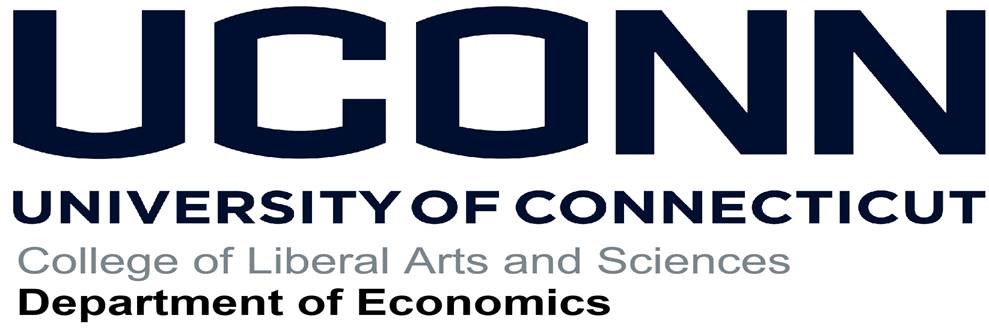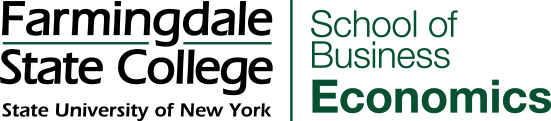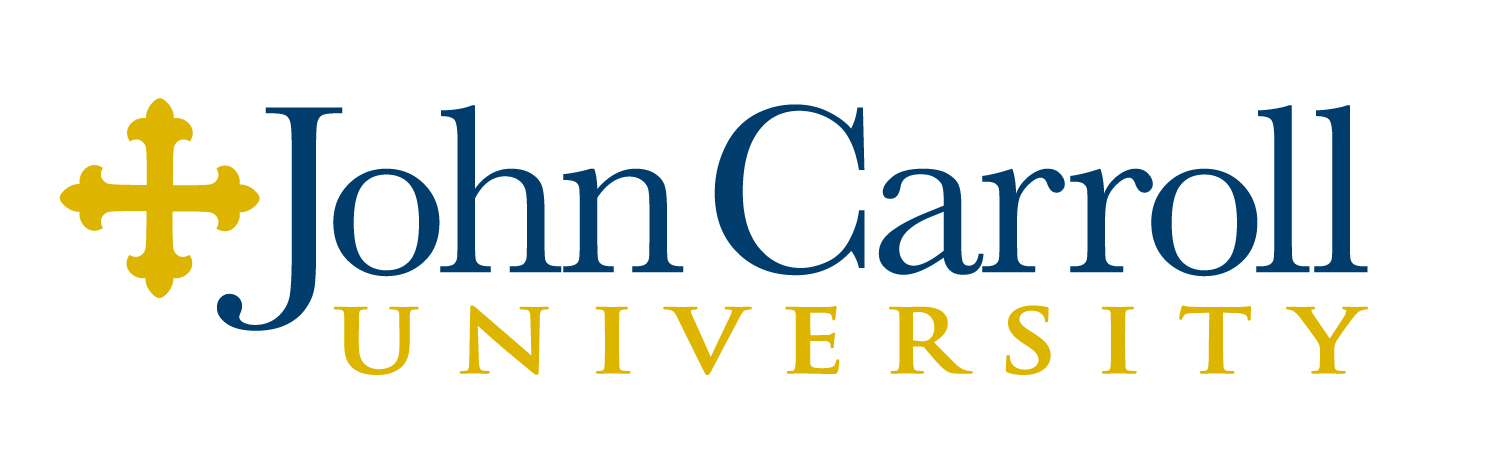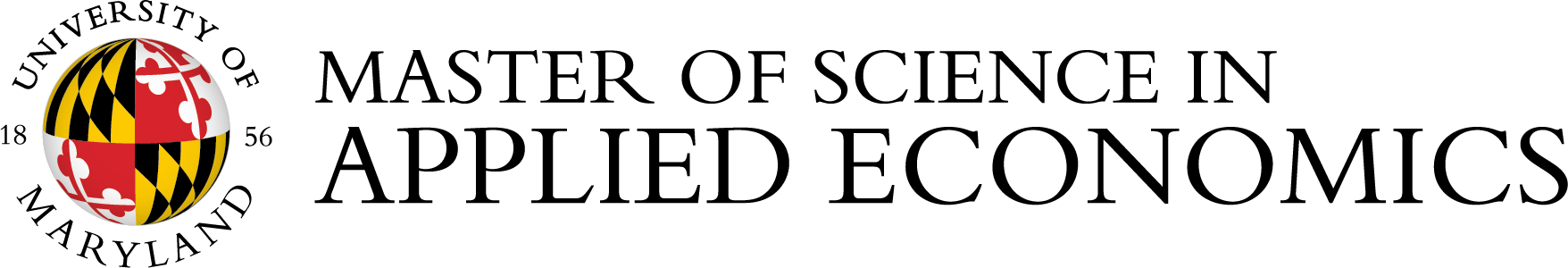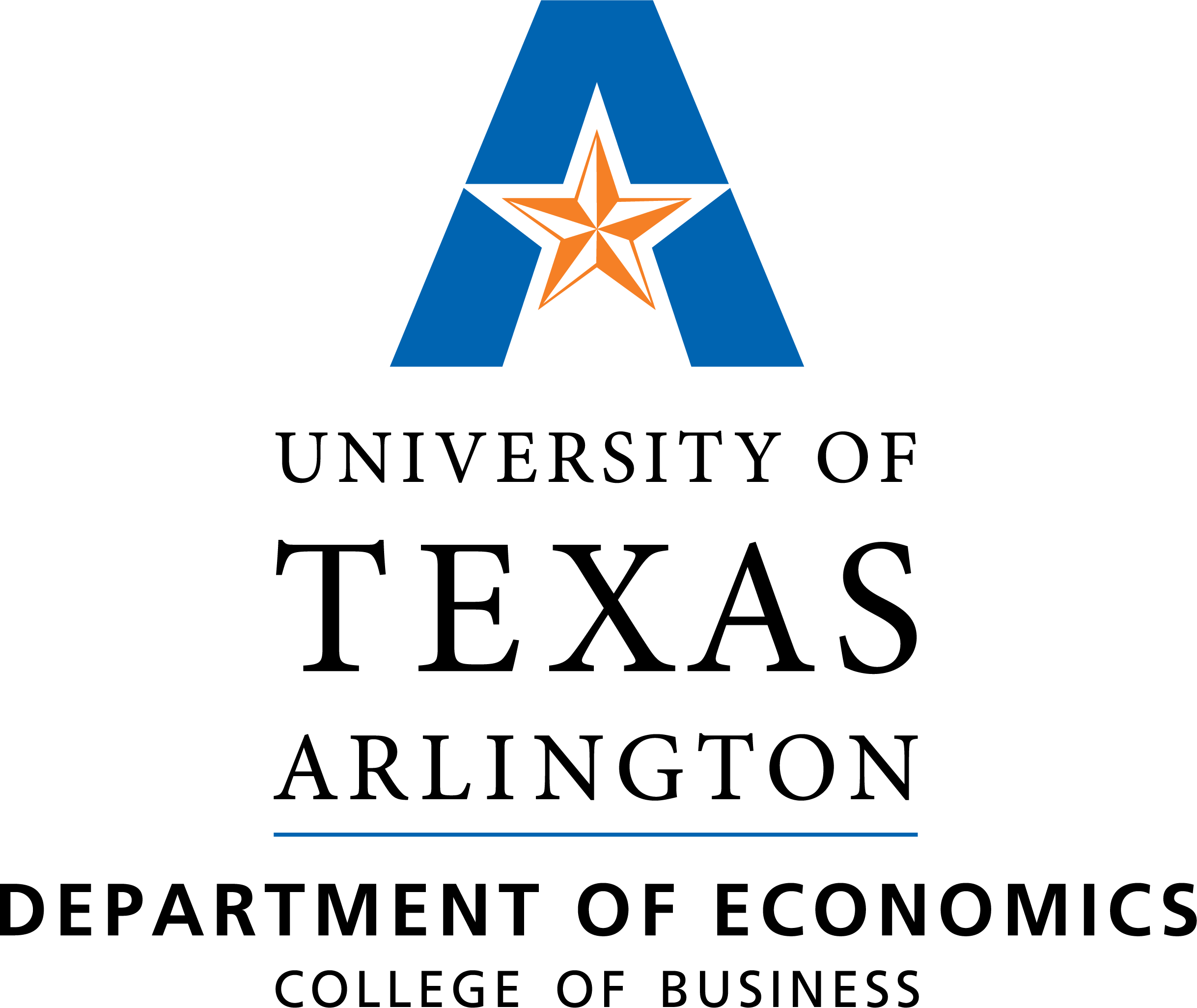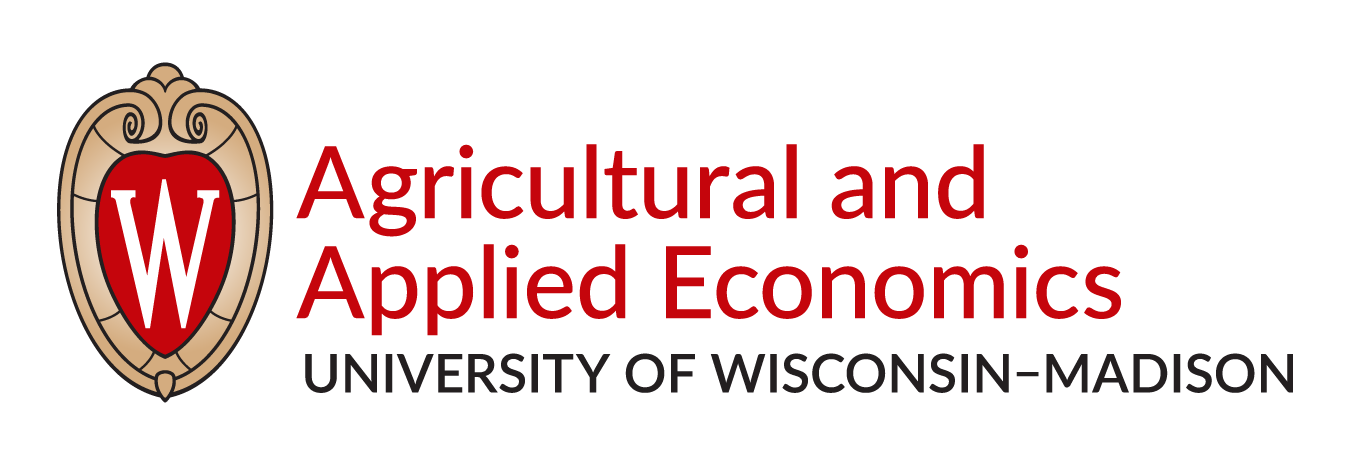Is your university interested in partnering with NABE on the CBE program?  There are many benefits to partnering with NABE to incorporate the CBE curriculum into university programs. More information